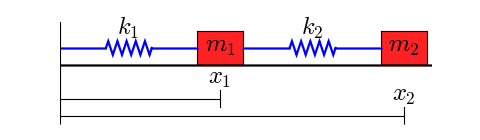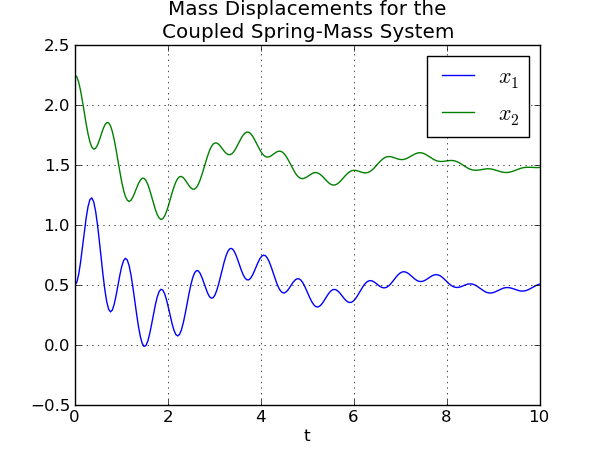This is an archival dump of old wiki content --- see scipy.org for current material.

This cookbook example shows how to solve a system of differential equations. (Other examples include the Lotka-Volterra Tutorial, the Zombie Apocalypse and the KdV example.)

## A Coupled Spring-Mass System

This figure shows the system to be modeled:Two objects with masses m1 and m2 are coupled through springs with spring constants k1 and k2. The left end of the left spring is fixed. We assume that the lengths of the springs, when subjected to no external forces, are L1 and L2.

The masses are sliding on a surface that creates friction, so there are two friction coefficients, b1 and b2.

The differential equations for this system are

m1 x1' ' + b1 x1' + k1 (x1 - L1) - k2 (x2 - x1 - L2) = 0

m2 x2' ' + b2 x2' + k2 (x2 - x1 - L2) = 0

This is a pair of coupled second order equations. To solve this system with one of the ODE solvers provided by SciPy, we must first convert this to a system of first order differential equations. We introduce two variables

y1 = x1', y2 = x2'

These are the velocities of the masses.

With a little algebra, we can rewrite the two second order equations as the following system of four first order equations:

x1' = y1

y1' = (-b1 y1 - k1 (x1 - L1) + k2 (x2 - x1 - L2))/m1

x2' = y2

y2' = (-b2 y2 - k2 (x2 - x1 - L2))/m2

These equations are now in a form that we can implement in Python.

The following code defines the "right hand side" of the system of equations (also known as a vector field). I have chosen to put the function that defines the vector field in its own module (i.e. in its own file), but this is not necessary. Note that the arguments of the function vectorfield are configured to be used with the odeint function: the time t is the second argument.

```   1 #
2 # two_springs.py
3 #
4 """
5 This module defines the vector field for a spring-mass system
6 consisting of two masses and two springs.
7 """
8
9
10 def vectorfield(w, t, p):
11     """
12     Defines the differential equations for the coupled spring-mass system.
13
14     Arguments:
15         w :  vector of the state variables:
16                   w = [x1,y1,x2,y2]
17         t :  time
18         p :  vector of the parameters:
19                   p = [m1,m2,k1,k2,L1,L2,b1,b2]
20     """
21     x1, y1, x2, y2 = w
22     m1, m2, k1, k2, L1, L2, b1, b2 = p
23
24     # Create f = (x1',y1',x2',y2'):
25     f = [y1,
26          (-b1 * y1 - k1 * (x1 - L1) + k2 * (x2 - x1 - L2)) / m1,
27          y2,
28          (-b2 * y2 - k2 * (x2 - x1 - L2)) / m2]
29     return f
```

Next, here is a script that uses odeint to solve the equations for a given set of parameter values, initial conditions, and time interval. The script prints the points in the solution to the terminal. Normally you will redirect its output to a file.

```   1 #
2 # two_springs_solver.py
3 #
4 """Use ODEINT to solve the differential equations defined by the vector field
5 in two_springs.py.
6 """
7
8 from scipy.integrate import odeint
9 import two_springs
10
11 # Parameter values
12 # Masses:
13 m1 = 1.0
14 m2 = 1.5
15 # Spring constants
16 k1 = 8.0
17 k2 = 40.0
18 # Natural lengths
19 L1 = 0.5
20 L2 = 1.0
21 # Friction coefficients
22 b1 = 0.8
23 b2 = 0.5
24
25 # Initial conditions
26 # x1 and x2 are the initial displacements; y1 and y2 are the initial velocities
27 x1 = 0.5
28 y1 = 0.0
29 x2 = 2.25
30 y2 = 0.0
31
32 # ODE solver parameters
33 abserr = 1.0e-8
34 relerr = 1.0e-6
35 stoptime = 10.0
36 numpoints = 250
37
38 # Create the time samples for the output of the ODE solver.
39 # I use a large number of points, only because I want to make
40 # a plot of the solution that looks nice.
41 t = [stoptime * float(i) / (numpoints - 1) for i in range(numpoints)]
42
43 # Pack up the parameters and initial conditions:
44 p = [m1, m2, k1, k2, L1, L2, b1, b2]
45 w0 = [x1, y1, x2, y2]
46
47 # Call the ODE solver.
48 wsol = odeint(two_springs.vectorfield, w0, t, args=(p,),
49               atol=abserr, rtol=relerr)
50
51 # Print the solution.
52 for t1, w1 in zip(t, wsol):
53     print t1, w1, w1, w1, w1
```

The following script uses Matplotlib to plot the solution generated by two_springs_solver.py

```   1 #
2 # two_springs_plot.py
3 #
4 """Plot the solution that was generated by two_springs_solver.py."""
5
7 from pylab import figure, plot, xlabel, grid, hold, legend, title, savefig
8 from matplotlib.font_manager import FontProperties
9
10
11 t, x1, xy, x2, y2 = loadtxt('two_springs.dat', unpack=True)
12
13 figure(1, figsize=(6, 4.5))
14
15 xlabel('t')
16 grid(True)
17 hold(True)
18 lw = 1
19
20 plot(t, x1, 'b', linewidth=lw)
21 plot(t, x2, 'g', linewidth=lw)
22
23 legend((r'\$x_1\$', r'\$x_2\$'), prop=FontProperties(size=16))
24 title('Mass Displacements for the\nCoupled Spring-Mass System')
25 savefig('two_springs.png', dpi=100)
```

The commands

```python two_springs_solver.py > two_springs.dat
python two_springs_plot.py ```

generate the following plot of the solution:SciPy: Cookbook/CoupledSpringMassSystem (last edited 2015-10-24 17:48:24 by anonymous)